# Plus Two Physics Notes Chapter 12 Atoms

Students can Download Chapter 12 Atoms Notes, Plus Two Physics Notes helps you to revise the complete Kerala State Syllabus and score more marks in your examinations.

## Kerala Plus Two Physics Notes Chapter 12 Atoms

Introduction
What is the arrangement of +ve charge and the electrons inside the atom? In other words, what is the structure of an atom?

Alpha-particle Scattering And Rutherford’s Nuclear Model Of Atom
Rutherford’s scattering experiment: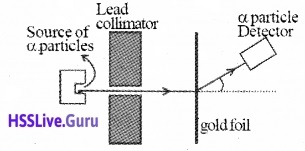Experimental arrangement:
α particles are incident on a gold foil (very small thickness) through a lead collimator. They are scattered at different angles. The scattered particles are counted by a particle detector.

Observations:
Most of the alpha particles are scattered by small angles. A few alpha particles are scattered at an angle greater than 90°.Conclusions

1. Major portion of the atom is empty space.
2. All the positive charges of the atom are concentrated in a small portion of the atom.
3. The whole mass of the atom is concentrated in a small portion of the atom.

Rutherford’s model of atom

1. The massive part of the atom (nucleus) is concentrated at the centre of the atom.
2. The nucleus contains all the positive charges of the atom.
3. The size of the nucleus is the order of 10-15m.
4. Electrons move around the nucleus in circular orbits.
5. The electrostatic force of attraction (between proton and electron) provides centripetal force.

1. Alpha-particle trajectory and Impact parameter:
The impact parameter is the perpendicular distance of the initial velocity vector of the a particle from the centre of the nucleus.It is seen that an α particle close to the nucleus (small impact parameter) suffers large scattering. In case of head-on collision, the impact parameter is minimum and the α particle rebounds back. For a large impact parameter, the α particle goes nearly undeviated and has a small deflection.

2. Electron orbits (Rutherford model of atom):
In Rutherford atom model, electrons are revolving around the positively charged nucleus. The electro-static force of attraction between the positive charge and negative charge provide centripetal force required for rotation.
For a dynamically stable orbit,
Centripetal force = Electrostatic force of attraction
Fc = FeThus the relation between the orbit radius and the electrons velocity,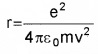Total energy of electron of Hydrogen atom (Rutherford model atom):
From eq. (1), we get∴ Kinetic energy of electron
KE = $$\frac{1}{2}$$mv2 ……….(3)
Substituting eq.(2) in eq. (3) we get
KE = $$\frac{e^{2}}{8 \pi \varepsilon_{0} r}$$ …………(4)
The electrostatic potential energy of hydrogen atom
$$\frac{e^{2}}{8 \pi \varepsilon_{0} r}$$
u = $$\frac{-e^{2}}{4 \pi \varepsilon_{0} r}$$ ………..(5)
∴ The total energy E of the electron in a hydrogen atom
E = K.E + Potential energy (U)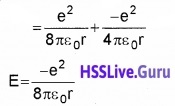The total energy of the electron is negative. This implies that the electron is bound to the nucleus.
If E is positive, the electron will escape from the nucleus.

Atomic Spectra
There are two types of spectra

1. Emission spectra
2. Absorption spectra

1. Emission spectra:
When an atomic gas or vapor is excited, the emitted radiation has a spectrum which contains certain wavelength only. A spectrum of this kind is termed as emission line spectrum. It consists of bright lines on a dark background.

Absorption spectra:
When white light passed through a gas, the transmitted light has spectrum contain certain wavelength only. A spectrum of this kind is termed as absorption line spectrum. It consists of dark lines on a bright background.

1. Spectral series: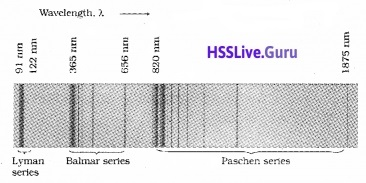The frequencies of the light emitted by a particular element exhibit some regular pattern. Hydrogen is the simplest atom and therefore, has the simplest spectrum, the spacing between lines of the hydrogen spectrum decreases in a regular way. Each of these sets is called a spectral series.

The first such series was observed by a Johann Jakob Balmer in the visible region of the hydrogen spectrum. This series is called Balmer series. Balmer found a simple empirical formula for the observed wavelengths.where λ is the wavelength, R is a constant called the Rydberg constant, and n may have integral values 3, 4, 5, etc. The value of R is 1.097 × 107m-1. This equation is also called Balmer formula.

Other series of spectra for hydrogen were discovered. These are known, as Lyman, Paschen, Brackett, and Pfund series. These are represented by the formulae:
Lyman series: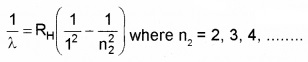Balmer series: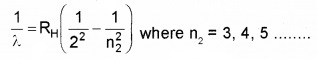Paschen series:
This series is in the infrared region. For this series the electron must jump from higher orbit to the third orbit.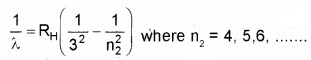Bracket series:
This series is the infrared region, for this the electron must jump from higher energy level to fourth orbit.P-fund series:
This series is in the infrared region.Bohr Model Of Hydrogen Atom
Limitations of Rutherford model:
1. Circular motion is an accelerated motion, an accelerated charge emit radiations. So that electron should emit radiation. Due to this emission of radiation, the energy of the electron decreases. Thus the atom becomes unstable.

2. There is no restriction for the radius of the orbit. So that electron can emit radiations of any frequency.

Bohr postulates:
Bohr combined classical and early quantum concepts and gave his theory in the form of three postulates.

• Electrons revolve round the positively charged nucleus in circular orbits.
• The electron which remains in a privileged path cannot radiate its energy.
• The orbital angular momentum of the electron is an integral multiple of h/π.
• Emission or Absorption of energy takes place when an electron jumps from one orbit to another.

Consider an electron of charge ‘e’ and mass m revolving round the positively charged nucleus in circular orbit of radius ‘r’. The force of attraction between the nucleus and the electron is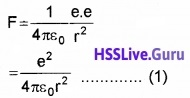This force provides the centripetal force for the orbiting electron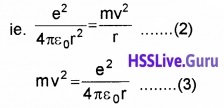According to Bohr’s second postulate, we can write
Angular momentum, mvr $$=\frac{n h}{2 \pi}$$.
ie. v = $$\frac{n h}{2 \pi m r}$$ _____(4)
Substituting this value of ‘v’ in equation (2), we get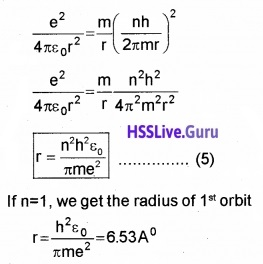Energy of the hydrogen atom:
The K.E. of revolving electron is
K.E$$=\frac{1}{2}$$ mv2 ______(6)
Substituting the value of equation (3) in eq.(6), we get
K.E = $$\frac{1}{2} \frac{e^{2}}{4 \pi \varepsilon_{0} r}$$ ______(7)
The potential energy of the electron,
P.E = $$\frac{-e^{2}}{4 \pi \varepsilon_{0} r}$$ _______(8)
ie. The Total energy of the hydrogen atom is,
T.E = Ke + PESubstituting the value of equation (5) in equation (9) we get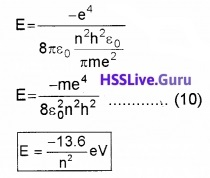1. Energy levels
Ground state (E1):
Ground state is the lowest energy state, in which the electron revolving in the orbit of smallest radius.
For ground state n = 1
∴ Energy of hydrogen atom E1 = $$\frac{-13.6}{n^{2}}$$ = -13.6 ev.

Excited State (E2):
When hydrogen atom receives energy, the electrons may raise to higher energy levels. Then atom is said to be in excited state.

First Excited state:
For first excited state n = 2
∴ Energy of first excited state E2 = $$\frac{-13.6}{2^{2}}$$ = -3.04ev
Similarly energy of second excited state
E3 = $$\frac{-13.6}{3^{2}}$$ = -1.51ev

Energy difference between E1 and E2 of H atom:
The energy required to exist an electron in hydrogen atom to its first existed state.
∆E = E2 – E1 = 3.4 – 13.6 = 10.2eV.

Ionization energy:
Ionization energy is the minimum energy required to free the electron from the ground state of atom. (ie. n = 1 to n = ∞)
The ionization of energy of hydrogen atom = 13.6 ev

2. Energy level diagram of hydrogen atom:Note:
An electron can have any total energy above E = 0ev. In such situations electron is free. Thus there is a continuum of energy states above E = 0ev.

The Line Spectra Of The Hydrogen Atom
According to the third postulate of Bohr’s model, when an atom makes a transition from higher energy state (ni) to lower energy state (nf), photon of energy hvif is emitted.
ie. hνif = Eni – EnfDe Broglie’s Explanation Of Bohr’s Second Postulate Of Quantization
Louis de Broglie argued that the electron in its circular orbit, behalf as a particle wave. Particle waves can produce standing waves under resonant conditions.
The condition to get standing wave,
2πrn = nλ
n = 1, 2, 3……..
The quantized electron orbits and energy states are due to the wave nature of the electron.

DeBroglie’s Proof for Bohr’s second postulate:
According to De Broglie, the electron in a circuit orbit is a particle wave. The particle wave can produce standing waves under resonant conditions. The condition for resonance for an electron moving in nth circular orbit of radius rn,
2πrn = nλ______(1)
n = 1, 2, 3………
If the speed of electron is much less than the speed of light, wave length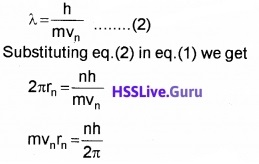Note:
The quantized electron orbits and energy states are due to the wave nature of the electron.

Limitations of Bohr atom model:

1. The Bohr model is applicable to hydrogenic atoms. It cannot be extended to many electron atoms such as helium
2. The model is unable to explain the relative intensities of the frequencies in the spectrum.
3. Bohr model could not explain fine structure of spectral lines.
4. Bohr theory could not give a satisfactory explanation for circular orbit.